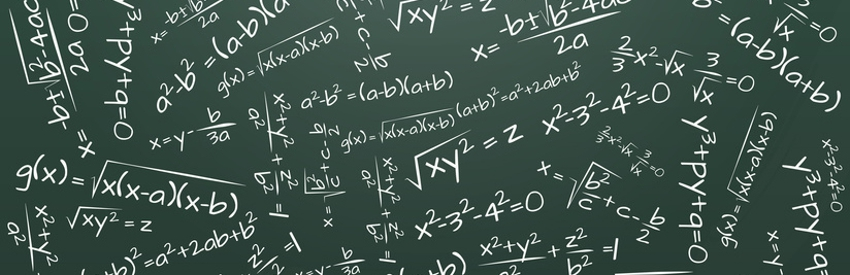# Mathematics

To encourage pupils to aim high and to work hard to achieve this, to develop pupils’ confidence in using Mathematics, to teach pupils how to calculate; mentally, using paper and pencil and using calculators and computers, to allow pupils to investigate, to make generalisations and to solve problems and to emphasise the practical nature of Mathematics and to help pupils understand the importance of Mathematics in modern life.

National 3: Mathematics
There are 3 units: -
• Manage Money and Data
• Shape, Space and Measures
• Numeracy
Each unit is internally assessed on a pass/fail basis.

National 4: Mathematics
There are 3 units: -
• Expressions and Formulae
• Relationships
• Numeracy
Units are internally assessed. Overall pass/fail is awarded when Added Value Test is completed.

National 5: Mathematics
There are 3 units: -
• Expressions and Formulae
• Relationships
• Applications
Each unit is internally assessed on a pass/fail basis.

National 5: Assessment
This course assessment has 2 components:
Component 1: question paper, paper 1 (non-calculator), 50 marks, 1 hour and 15 minutes.
Component 2: question paper, paper 2, 60 marks, 1 hour and 50 minutes.

National 4: Lifeskills Mathematics
There are 3 units: -
• Managing Finance and Statistics
• Geometry and Measures
• Numeracy
Units are internally assessed. Overall pass/fail is awarded when Added Value Test is completed.

PROGRESSION PATHS
• Linear progression – A quality pass at National 5, can lead to studying Higher Mathematics. A quality pass at Higher can lead to studying Advanced Higher Mathematics.
• Skills progression – Learning mathematics develops logical reasoning, analysis, problem solving skills, creativity, and the ability to think in abstract ways. It uses a universal language of numbers and symbols, which allows us to communicate ideas in a concise, unambiguous and rigorous way across the curriculum.

HIGHER
There are 3 units:-
• Unit 1: Expressions and Functions: - logarithmic and exponential functions, trigonometric expressions and vectors.
• Unit 2: Relationships and Calculus: - algebraic and trigonometric equations and calculus.
• Unit 3: Applications: - straight line, circles, sequences and calculus in context. Entry to this course requires National 5 skills, knowledge and understanding. Each unit will be internally assessed on a pass/fail basis.
EXTERNAL ASSESSMENT There are 2 papers:-
• Paper 1 (non-calculator) 70 minutes, 60 marks.
• Paper 2 (calculator) 90 minutes, 70 marks.
To gain an award, candidates must pass both the internal unit tests and the external assessment.

ADVANCED HIGHER MATHEMATICS, MECHANICS and STATISTICS
• All units are internally assessed.
• At the end of each unit you will sit a unit test and be awarded a pass/fail.
• The final exam lasts 3 hours, is worth100 marks and is graded A – D.
• A calculator may be used for all unit assessments and the final assessment.
• To gain Advanced Higher you must pass all 3 units and the final exam (additionally you must carry out a statistical investigation for Advanced Higher Statistics)
Methods in Algebra and Calculus (Advanced Higher)
• use mathematical operational skills linked to methods in algebra and calculus
Applications of Algebra and Calculus (Advanced Higher)
• use mathematical operational skills linked to applications of algebra and calculus
• use mathematical reasoning skills linked to applications of algebra and calculus
Geometry, Proof and Systems of Equations (Advanced Higher)
• use mathematical operational skills linked to geometry, proof and systems of equations
Mathematics of Mechanics: Linear and Parabolic Motion (Advanced Higher)
• use mathematical operational and reasoning skills linked to linear and parabolic motion
• Mathematics of Mechanics: Force Energy and Periodic Motion (Advanced Higher)
• use mathematical operational and reasoning skills linked to force, energy and periodic motion
Mathematics of Mechanics: Mathematical Techniques for Mechanics (Advanced Higher)
• use mathematical operational and reasoning skills linked to mechanics